JS数据结构:树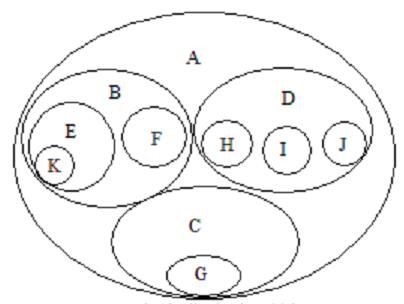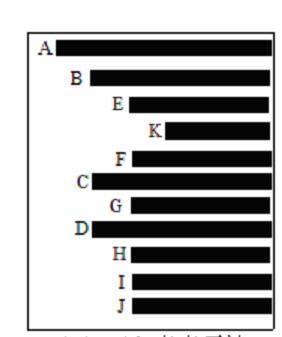3.广义表表示法

(A(B(E(k),F),C(G),D(H,I,J))

4.树形表示法 （最常见的表示法）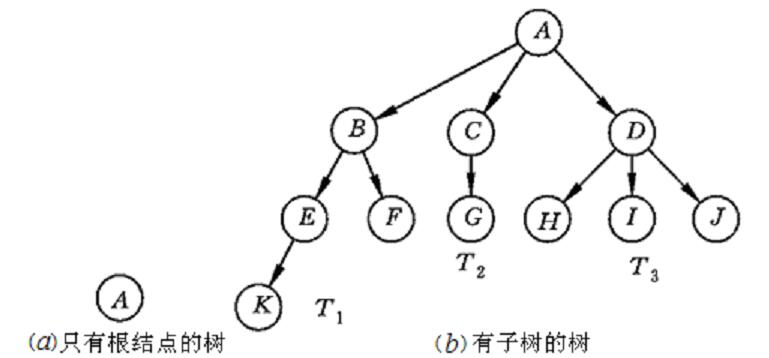1.实现二叉查找树

function Node(data, left, right) {
this.data = data;
this.left = left;
this.right = right;
this.show = show;
}

function show() {
return this.data;
}

function insert(data) {
var n = new Node(data, null, null);
if (!this.root) {
this.root = n;
} else {
var current = this.root, parent;
while (true) {
parent = current;
if (data < current.data) {
current = current.left;
if (!current) {
parent.left = n;
break;
}
} else {
current = current.right;
if (!current) {
parent.right = n;
break;
}
}
}
}
} 

function BST () {
this.root = null;
this.insert = insert;
this.inOrder = inOrder;
this.preOrder = preOrder;
this.postOrder = postOrder;
}

//中序遍历
function inOrder (node) {
if (node) {
inOrder(node.left);
console.log(node.show());
inOrder(node.right);
}
}

//先序遍历
function preOrder (node) {
if (node) {
console.log(node.show());
preOrder(node.left);
preOrder(node.right);
}
}

//后序遍历
function postOrder (node) {
if (node) {
postOrder(node.left);
postOrder(node.right);
console.log(node.show());
}
}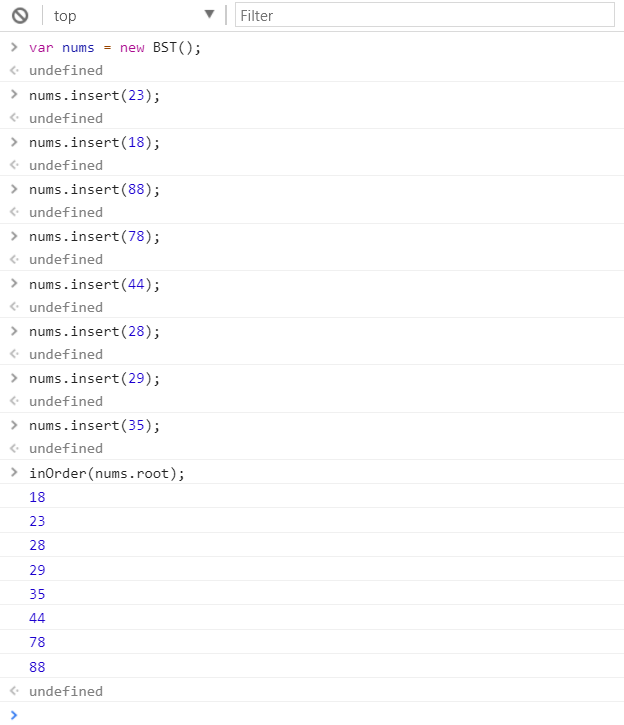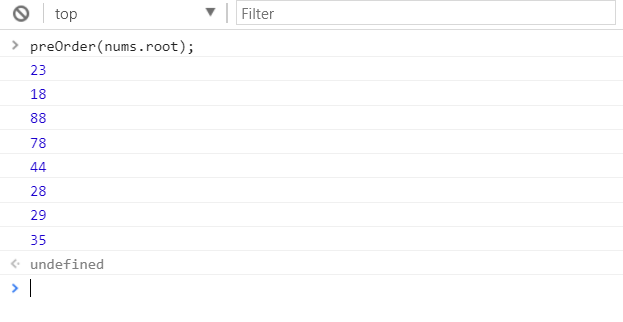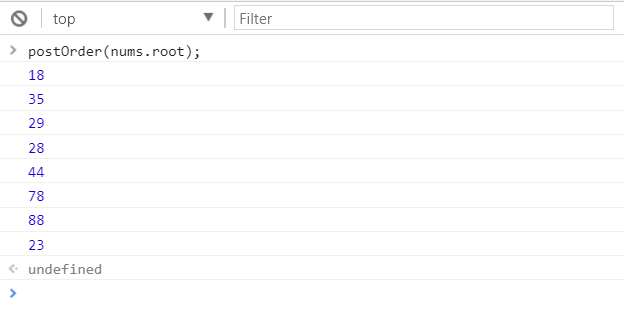1.查找给定值

2.查找最大值

3.查找最小值

function getMin () {
var current =nums.root;
while (current.left) {
current = current.left;
}
return current.data;
}

function getMax () {
var current = nums.root;
while (current.right) {
current = current.right;
}
return current.data;
}

function find (data) {
var current = nums.root;
while (current) {
if (current.data === data) {
return current;
} else if (data < current.data) {
current = current.left;
} else {
current = current.right;
}
}
return null;
}

* 注意 上面3个函数中引用了我的创建的变量 nums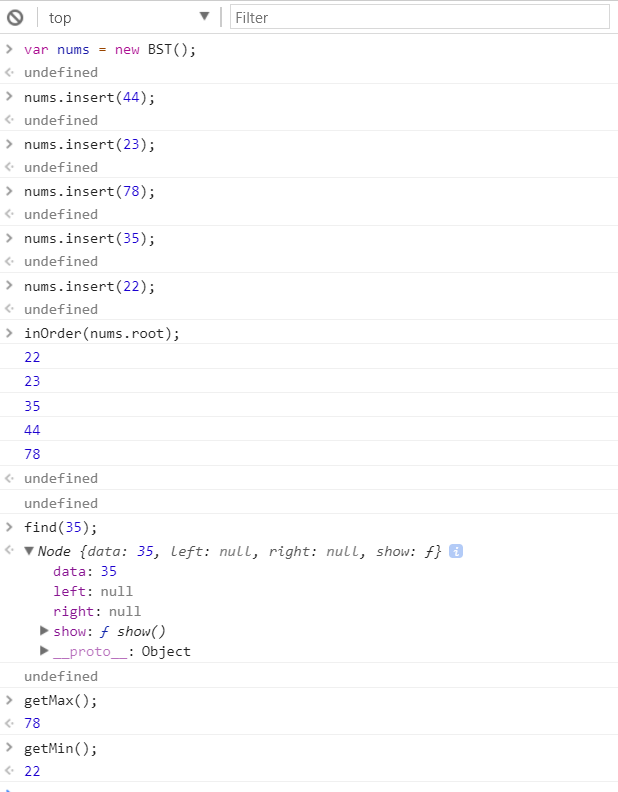如果待删除的节点是叶子节点，那么只需要将从父节点指向它的连接指向null；如果待删除的节点只包含一个子节点，那么原本指向它的节点就得做些调整，使其指向它的子节点；如果待删除的节点包含两个子节点，正确的做法有两种：要么查找待删除的节点左子树上的最大值，要么查找其右子树上的最小值。这里我选用后一种方式。

function removeNode (node, data) {
if (!node) {
return null;
}
if (data === node.data) {
//没有子节点
if ((!node.left) && (!node.right)) {
return null;
}
//没有左子节点的节点
if (!node.left) {
return node.right;
}
//没有右子节点
if (!node.right) {
return node.left;
}
//有两个子节点的节点

//这里先拿到右子树上的最小值的节点，
var tempNode = getSmallest (node.right);
node.data = tempNode.data;
node.right = removeNode(node.right, tempNode.data);
return node;
} else if (data < node.data) {
node.left = removeNode(node.left, data);
return node;
} else {
node.right = removeNode(node.right, data);
return node;
}
}